# Magnetic fields in circular loop

• Biker

#### Biker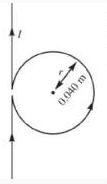If I have a some a wire and I took part of it and created a circle. If I want to calculate the strength of the magnetic field at the center of it. I should just use the magnetic field equation for the circular loop, shouldn't I?
## B = \frac{U_o N I}{2R} ##
and I shouldn't care about the intersection between the circle and the wire itself because the equation will include that point and the wire doesn't have an effect on the center of the circle.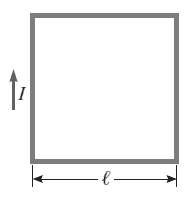And why if I want the magnetic field at the center this equation doesn't work?
##B = 4 ( \frac{U_O I}{ 2 \pi d} )##
I have seen a webpage before that uses calculus in order to solve this (Havent taken it yet) But what does calculus has to do in this shape? Reference: http://www.physicspages.com/2013/04/11/magnetic-field-of-square-current-loop/

Last edited: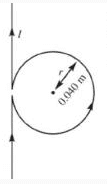If I have a some a wire and I took part of it and created a circle. If I want to calculate the strength of the magnetic field at the center of it. I should just use the magnetic field equation for the circular loop, shouldn't I?

No, you also have a contribution from the straight sections. The fields from the loop and the straight sections superpose (add). If the gap at the "intersection" is very small, you can treat the straight sections as if they were a single continuous wire.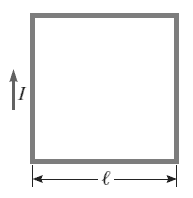And why if I want the magnetic field at the center this equation doesn't work?
##B = 4 ( \frac{U_O I}{ 2 \pi d} )##

That equation (the part in parentheses) is for an infinitely long straight wire.

I have seen a webpage before that uses calculus in order to solve this (Havent taken it yet) But what does calculus has to do in this shape? Reference: http://www.physicspages.com/2013/04/11/magnetic-field-of-square-current-loop/

To find the field from a finitely long straight wire, you have to integrate (add up) the contributions from each little piece of the wire. If you let the length of the wire approach infinity, you get the equation you gave above as the limiting case.

No, you also have a contribution from the straight sections. The fields from the loop and the straight sections superpose (add). If the gap at the "intersection" is very small, you can treat the straight sections as if they were a single continuous wire.

That equation (the part in parentheses) is for an infinitely long straight wire.

To find the field from a finitely long straight wire, you have to integrate (add up) the contributions from each little piece of the wire. If you let the length of the wire approach infinity, you get the equation you gave above as the limiting case.

In the place where I created the circle, I shouldn't count it as a straight wire and add it to the circle's MF. Because that small segment is already part of the circle so why should I add it twice ._.?

I'm not referring to the intersection point. I'm referring to the whole straight wire. The field at the center of the circle is the sum of the fields from the circle and from the straight wire.

I'm not referring to the intersection point. I'm referring to the whole straight wire. The field at the center of the circle is the sum of the fields from the circle and from the straight wire.
Sorry I still don't get what you mean.

I have solved question before about adding up the straight wire and circle MF but it was usually formed with two different wires. However, here it is only one and what is confusing me is that small gap. If I use the circle equation then I am saying that it is a full circle and when I use the straight wire equation I am saying that it is continuous but as you said we can assume that the gap is really small. But isn't there any intersection between the circle and the wire so I am probably adding up the strength of MF of a small piece twice using the both equation?

I have solved question before about adding up the straight wire and circle MF but it was usually formed with two different wires.
It makes no difference, provided that in the "two different wires" case the two wires carry the same amount of current.

In the "single wire" case, there is a point where the wire crosses over itself. In the "two different wires" case the two wires also have to overlap at one point, in order to make the two cases exactly similar.

It makes no difference, provided that in the "two different wires" case the two wires carry the same amount of current.

In the "single wire" case, there is a point where the wire crosses over itself. In the "two different wires" case the two wires also have to overlap at one point, in order to make the two cases exactly similar.
...
I didn't mean that. I should have mentioned "Two wires not touching each other"

All I want is an explanation of this:

If I use the circle equation then I am saying that it is a full circle and when I use the straight wire equation I am saying that it is continuous but as you said we can assume that the gap is really small. But isn't there any intersection between the circle and the wire so I am probably adding up the strength of MF of a small piece twice using the both equation?

Something like this: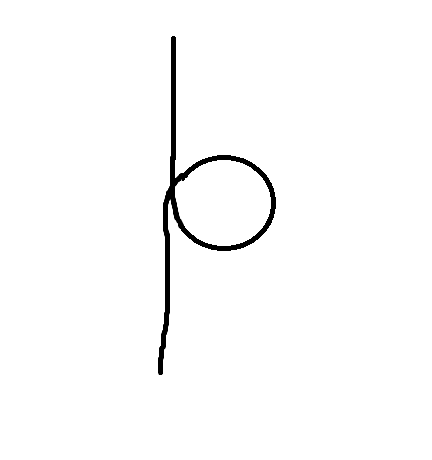But isn't there any intersection between the circle and the wire so I am probably adding up the strength of MF of a small piece twice using the both equation?
I'm saying that in both cases, you actually have two small pieces of wire, overlapping at the intersection point. So of course you're going to have to include both of them when calculating the field. Finding the sum of the fields from the (whole) circle and from the (whole) long straight wire does just that!